# Fourier Analysis

## Producer Field Guide

HGD_Product
Producer Field Guide
HGD_Portfolio_Suite
Producer

Image enhancement techniques can be divided into two basic categories:

• Point
• Neighborhood

Point techniques enhance the pixel based only on its value, with no concern for the values of neighboring pixels. These techniques include contrast stretches (nonadaptive), classification, and level slices.

Neighborhood techniques enhance a pixel based on the values of surrounding pixels. As a result, these techniques require the processing of a possibly large number of pixels for each output pixel.

The most common way of implementing these enhancements is by using a moving window convolution. However, as the size of the moving window increases, the number of requisite calculations becomes enormous. An enhancement that requires a convolution operation in the spatial domain can be implemented as a simple multiplication in frequency space—a much faster calculation.

In ERDAS IMAGINE, the FFT calculation is used to convert a raster image from the spatial (normal) domain into a frequency domain image. The FFT calculation converts the image into a series of two-dimensional sine waves of various frequencies. The Fourier image itself cannot be easily viewed, but the magnitude of the image can be calculated, which can then be displayed either in the Viewer or in the FFT Editor. Analysts can edit the Fourier image to reduce noise or remove periodic features, such as striping. Once the Fourier image is edited, it is then transformed back into the spatial domain by using an IFFT. The result is an enhanced version of the original image.

This section focuses on Fourier editing techniques available in FFT Editor. Some rules and guidelines for using these tools are presented, as well as some examples of techniques that generally work for specific applications, such as striping.

The basic premise behind a Fourier transform is that any one-dimensional function, f(x) (which might be a row of pixels), can be represented by a Fourier series consisting of some combination of sine and cosine terms and their associated coefficients. For example, a line of pixels with a high spatial frequency gray scale pattern might be represented in terms of a single coefficient multiplied by a sin(x) function. High spatial frequencies are those that represent frequent gray scale changes in a short pixel distance. Low spatial frequencies represent infrequent gray scale changes that occur gradually over a relatively large number of pixel distances. A more complicated function, f(x), might have to be represented by many sine and cosine terms with their associated coefficients.

One-Dimensional Fourier Analysis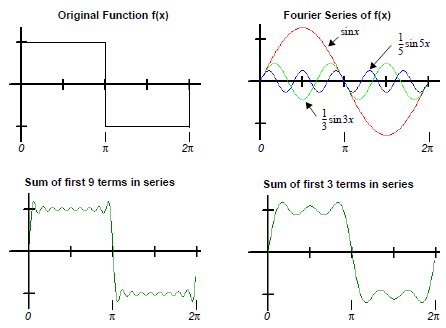The figure above shows how a function f(x) can be represented as a linear combination of sine and cosine. In this example the function is a square wave whose cosine coefficients are zero leaving only sine terms. The first three terms of the Fourier series are plotted in the upper right graph and the plot of the sum is shown below it. After nine iterations, the Fourier series is approaching the original function.

A Fourier transform is a linear transformation that calculates the coefficients necessary for the sine and cosine terms to adequately represent the image. This theory is used extensively in electronics and signal processing, where electrical signals are continuous and not discrete. Therefore, DFT has been developed. Because of the computational load in calculating the values for all the sine and cosine terms along with the coefficient multiplications, a highly efficient version of the DFT was developed and named the FFT.

To handle images that consist of many one-dimensional rows of pixels, a two-dimensional FFT has been devised that incrementally uses one-dimensional FFTs in each direction and then combines the result. These images are symmetrical about the origin.

Applications

Fourier transformations are typically used for the removal of noise such as striping, spots, or vibration in imagery by identifying periodicities (areas of high spatial frequency). Fourier editing can be used to remove regular errors in data such as those caused by sensor anomalies (for example, striping). This analysis technique can also be used across bands as another form of pattern or feature recognition.

FFT

The FFT calculation is: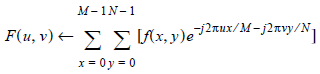Where:

M = number of pixels horizontally

N = number of pixels vertically

u,v = spatial frequency variables

e = 2.71828, the natural logarithm base

j = imaginary component of a complex number

The number of pixels horizontally and vertically must each be a power of two. If the dimensions of the input image are not a power of two, they are padded up to the next highest power of two. See Padding Technique.

Source: Modified from Oppenheim and Schafer, 1975; Press et al, 1988.

Images computed by this algorithm are saved with an .fft file extension.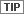You should run a Fourier Magnitude transform on an .fft file before viewing it in the Viewer. The FFT Editor displays the magnitude without further processing.

Fourier Magnitude

The raster image generated by the FFT calculation is not an optimum image for viewing or editing. Each pixel of a fourier image is a complex number (that is, it has two components: real and imaginary). For display as a single image, these components are combined in a root-sum of squares operation. Also, since the dynamic range of Fourier spectra vastly exceeds the range of a typical display device, the Fourier Magnitude calculation involves a logarithmic function.

Finally, a Fourier image is symmetric about the origin (u, v = 0, 0). If the origin is plotted at the upper left corner, the symmetry is more difficult to see than if the origin is at the center of the image. Therefore, in the Fourier magnitude image, the origin is shifted to the center of the raster array.

In this transformation, each .fft layer is processed twice. First, the maximum magnitude,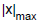, is computed. Then, the following computation is performed for each FFT element magnitude x: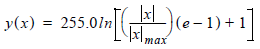Where:

x = input FFT element

y = normalized log magnitude of the FFT element= maximum magnitude

e = 2.71828, the natural logarithm base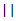= magnitude operator

This function was chosen so that y would be proportional to the logarithm of a linear function of x, with y(0)=0 and y () = 255.

In the figure below:

Image A is one band of a badly striped Landsat TM scene.

Image B is the Fourier Magnitude image derived from the Landsat image.

Example of Fourier Magnitude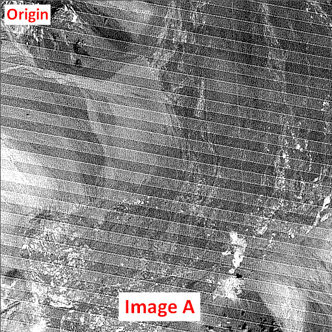Note that, although Image A has been transformed into Image B, these raster images are very different symmetrically. The origin of Image A is at (x, y) = (0, 0) in the upper left corner. In Image B, the origin (u, v) = (0, 0) is in the center of the raster. The low frequencies are plotted near this origin while the higher frequencies are plotted further out. Generally, the majority of the information in an image is in the low frequencies. This is indicated by the bright area at the center (origin) of the Fourier image.

It is important to realize that a position in a Fourier image, designated as (u, v), does not always represent the same frequency, because it depends on the size of the input raster image. A large spatial domain image contains components of lower frequency than a small spatial domain image. As mentioned, these lower frequencies are plotted nearer to the center (u, v = 0, 0) of the Fourier image. Note that the units of spatial frequency are inverse length, for example, m-1.

The sampling increments in the spatial and frequency domain are related by: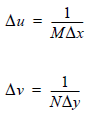Where:

M = horizontal image size in pixels

N = vertical image size in pixels

D x = pixel size

D y = pixel size

For example, converting a 512 × 512 Landsat TM image (pixel size = 28.5m) into a Fourier image: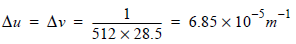u or v Frequency 0 0 1 6.85 × 10-5 × m-1 2 13.7 × 10-5 × m-1

If the Landsat TM image was 1024 × 1024: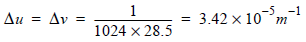u or v Frequency 0 0 1 3.42 × 10-5 × m-1 2 6.85 × 10-5 × m-1

So, as noted above, the frequency represented by a (u, v) position depends on the size of the input image.

For the above calculation, the sample images are 512 × 512 and 1024 × 1024 (powers of two). These were selected because the FFT calculation requires that the height and width of the input image be a power of two (although the image need not be square). In practice, input images usually do not meet this criterion. Three possible solutions are available in ERDAS IMAGINE:

• Subset the image.
• Pad the image—the input raster is increased in size to the next power of two by embedding it in a field of the mean value of the entire input image.
• Resample the image so that its height and width are powers of two.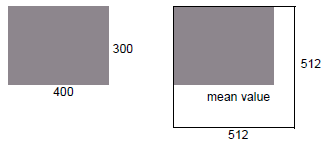The padding technique is automatically performed by the FFT program. It produces a minimum of artifacts in the output Fourier image. If the image is subset using a power of two (that is, 64 × 64, 128 × 128, 64 × 128), no padding is used.

IFFT

The IFFT computes the inverse two-dimensional FFT of the spectrum stored.

• The input file must be in the compressed .fft format described earlier (that is, output from the FFT or FFT Editor).
• If the original image was padded by the FFT program, the padding is automatically removed by IFFT.
• This program creates (and deletes, upon normal termination) a temporary file large enough to contain one entire band of .fft data.The specific expression calculated by this program is: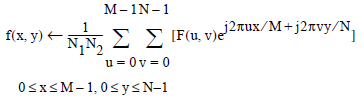Where:

M = number of pixels horizontally

N = number of pixels vertically

u, v = spatial frequency variables

e = 2.71828, the natural logarithm base

Source: Modified from Oppenheim and Schafer, 1975 and Press et al, 1988.

Images computed by this algorithm are saved with an .ifft.img file extension by default.

Filtering

Operations performed in the frequency (Fourier) domain can be visualized in the context of the familiar convolution function. The mathematical basis of this interrelationship is the convolution theorem, which states that a convolution operation in the spatial domain is equivalent to a multiplication operation in the frequency domain: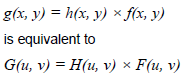Where:

f(x, y) = input image

h(x, y) = position invariant operation (convolution kernel)

g(x, y) = output image

G, F, H = Fourier transforms of g, f, h

The names high-pass, low-pass, high-frequency indicate that these convolution functions derive from the frequency domain.

Low-Pass Filtering

The simplest example of this relationship is the low-pass kernel. The name, low-pass kernel, is derived from a filter that would pass low frequencies and block (filter out) high frequencies. In practice, this is easily achieved in the spatial domain by the M = N = 3 kernel: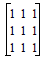Obviously, as the size of the image and, particularly, the size of the low-pass kernel increases, the calculation becomes more time-consuming. Depending on the size of the input image and the size of the kernel, it can be faster to generate a low-pass image via Fourier processing.

The figure below compares Direct and Fourier domain processing for finite area convolution.

Comparison of Direct and Fourier Domain Processing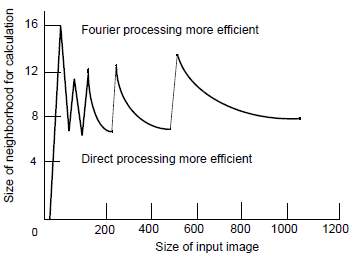Source: Pratt, 1991

In the Fourier domain, the low-pass operation is implemented by attenuating the pixels’ frequencies that satisfy: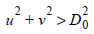D0 is often called the cutoff frequency.

As mentioned, the low-pass information is concentrated toward the origin of the Fourier image. Thus, a smaller radius (r) has the same effect as a larger N (where N is the size of a kernel) in a spatial domain low-pass convolution.

As was pointed out earlier, the frequency represented by a particular u, v (or r) position depends on the size of the input image. Thus, a low-pass operation of r = 20 is equivalent to a spatial low-pass of various kernel sizes, depending on the size of the input image.

For example:

 Image Size Fourier Low-Passr = Convolution Low-PassN = 64 × 64 50 3 30 3.5 20 5 10 9 5 14 128 × 128 20 13 10 22 256 × 256 20 25 10 42

This table shows that using a window on a 64 × 64 Fourier image with a radius of 50 as the cutoff is the same as using a 3 × 3 low-pass kernel on a 64 × 64 spatial domain image.

High-Pass Filtering

Just as images can be smoothed (blurred) by attenuating the high-frequency components of an image using low-pass filters, images can be sharpened and edge-enhanced by attenuating the low-frequency components using high-pass filters. In the Fourier domain, the high-pass operation is implemented by attenuating the pixels’ frequencies that satisfy: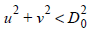Windows

The attenuation discussed above can be done in many different ways. In ERDAS IMAGINE Fourier processing, five window functions are provided to achieve different types of attenuation:

• Ideal Window
• Bartlett (triangular)
• Butterworth, Gaussian, and Hanning (cosine)

Each of these windows must be defined when a frequency domain process is used. This application is perhaps easiest understood in the context of the high-pass and low-pass filter operations. Each window is discussed in more detail:

Ideal Window

The simplest low-pass filtering is accomplished using the ideal window, so named because its cutoff point is absolute. Note that in the figure below the cross section is ideal.

Ideal Window Cross Section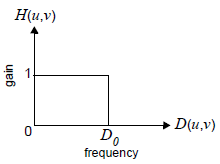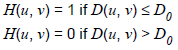All frequencies inside a circle of a radius D0 are retained completely (passed), and all frequencies outside the radius are completely attenuated. The point D0 is termed the cutoff frequency.

High-pass filtering using the ideal window looks like the following illustration:

High-Pass Filtering Using Ideal Window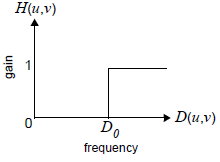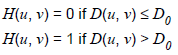All frequencies inside a circle of a radius D0 are completely attenuated, and all frequencies outside the radius are retained completely (passed).

A major disadvantage of the ideal filter is that it can cause ringing artifacts, particularly if the radius (r) is small. The smoother functions (for example, Butterworth and Hanning) minimize this effect.

Bartlett

Filtering using the Bartlett window is a triangular function, as shown in the following low- and high-pass cross sections:

Filtering Using Bartlett Window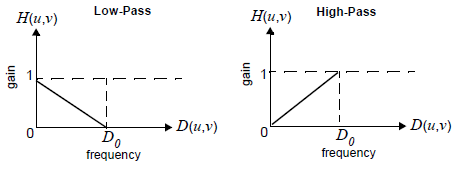Butterworth, Gaussian, and Hanning

Butterworth, Gaussian, and Hanning windows are all smooth and greatly reduce the effect of ringing. The differences between them are minor and are of interest mainly to experts. For most normal types of Fourier image enhancement, they are essentially interchangeable.

Butterworth window reduces the ringing effect because it does not contain abrupt changes in value or slope. The following low- and high-pass cross sections illustrate this:

Filtering Using Butterworth Window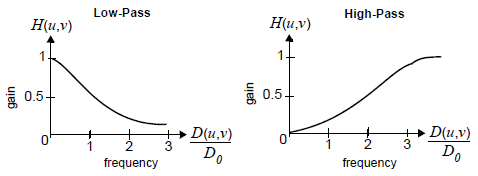The equation for low-pass Butterworth window is: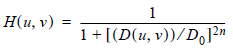The Butterworth window approaches its window center gain asymptotically.

The equation for Gaussian low-pass window is: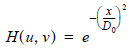The equation for Hanning low-pass window is: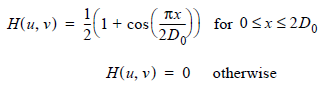Fourier Noise Removal

Occasionally, images are corrupted by noise that is periodic in nature. An example of this is the scan lines that are present in some TM images. When these images are transformed into Fourier space, the periodic line pattern becomes a radial line. The Fourier Analysis functions provide two main tools for reducing noise in images:

• editing
• automatic removal of periodic noise

Editing

In practice, it has been found that radial lines centered at the Fourier origin (u, v = 0, 0) are best removed using back-to-back wedges centered at (0, 0). It is possible to remove these lines using very narrow wedges with the Ideal window. However, the sudden transitions resulting from zeroing-out sections of a Fourier image causes a ringing of the image when it is transformed back into the spatial domain. This effect can be lessened by using a less abrupt window, such as Butterworth.

Other types of noise can produce artifacts, such as lines not centered at u,v = 0,0 or circular spots in the Fourier image. These can be removed using the tools provided in FFT Editor. As these artifacts are always symmetrical in the Fourier magnitude image, editing tools operate on both components simultaneously. Use FFT Editor tools to attenuate a circular or rectangular region anywhere on the image.

Automatic Periodic Noise Removal

Use FFT Editor to selectively and accurately remove periodic noise from any image. However, operator interaction and a bit of trial and error are required. The automatic periodic noise removal algorithm has been devised to address images degraded uniformly by striping or other periodic anomalies. Using this algorithm requires minimum information from you.

The image is first divided into 128 × 128 pixel blocks. The Fourier Transform of each block is calculated and the log-magnitudes of each FFT block are averaged. The averaging removes all frequency domain quantities except those that are present in each block (that is, some sort of periodic interference). The average power spectrum is then used as a filter to adjust the FFT of the entire image. When IFFT is performed, the result is an image that should have any periodic noise eliminated or significantly reduced. This method is partially based on the algorithms outlined in Cannon (Cannon, 1983) and Srinivasan et al (Srinivasan et al, 1988).

Select Periodic Noise Removal option from Radiometric Enhancement to use this function.

Homomorphic Filtering

Homomorphic filtering is based upon the principle that an image may be modeled as the product of illumination and reflectance components: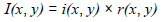Where:

I(x, y) = image intensity (DN) at pixel x, y

i(x, y) = illumination of pixel x, y

r(x, y) = reflectance at pixel x, y

The illumination image is a function of lighting conditions and shadows. The reflectance image is a function of the object being imaged. Use a log function to separate the two components (i and r) of the image: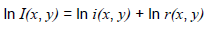This transforms the image from multiplicative to additive superposition. With the two component images separated, any linear operation can be performed. In this application, the image is now transformed into Fourier space. Because the illumination component usually dominates the low frequencies, while the reflectance component dominates the higher frequencies, the image may be effectively manipulated in the Fourier domain.

By using a filter on the Fourier image, which increases the high-frequency components, the reflectance image (related to the target material) may be enhanced, while the illumination image (related to the scene illumination) is de-emphasized.

Select Homomorphic Filter option in Spatial Enhancement to use this function.

By applying an IFFT followed by an exponential function, the enhanced image is returned to the normal spatial domain. The flow chart in the figure below summarizes the homomorphic filtering process in ERDAS IMAGINE.

Homomorphic Filtering Process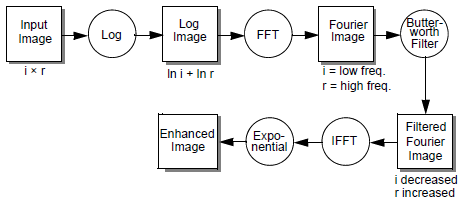As mentioned earlier, if an input image is not a power of two, ERDAS IMAGINE Fourier analysis automatically pads the image to the next largest size to make it a power of two. For manual editing, this causes no problems. However, in automatic processing, such as the homomorphic filter, the artifacts induced by the padding may have a deleterious effect on the output image. For this reason, it is recommended that images that are not a power of two be subset before being used in an automatic process.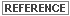A detailed description of the theory behind Fourier series and Fourier transforms is given in Gonzales and Wintz (Gonzalez and Wintz, 1977). See also Oppenheim (Oppenheim and Schafer, 1975) and Press (Press et al, 1988).